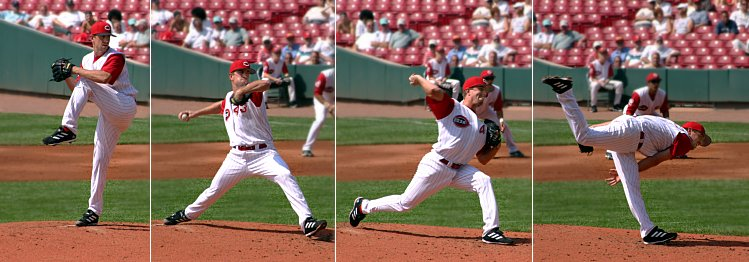# Impulse

(diff) ← Older revision | Latest revision (diff) | Newer revision → (diff)

Impulse is a change in momentum. Mathematically impulse is:

Whenever a force (F) is exerted for an interval of time (, often for a very short interval of time) momentum is changed. Impulses are a useful way of looking at average forces in collisions (which often, but not always, take very little time). In the context of energy science, this concept is often applied to specific turbines used in hydropower like crossflow turbines. In cases like this the impulse can be thought of as the force of the water on the water wheel.Figure 1. A pitcher gives an impulse to a baseball in order to increase its kinetic energy. His arm goes back as far as possible and then as forward as possible in order to maximize the time the force was applied.